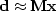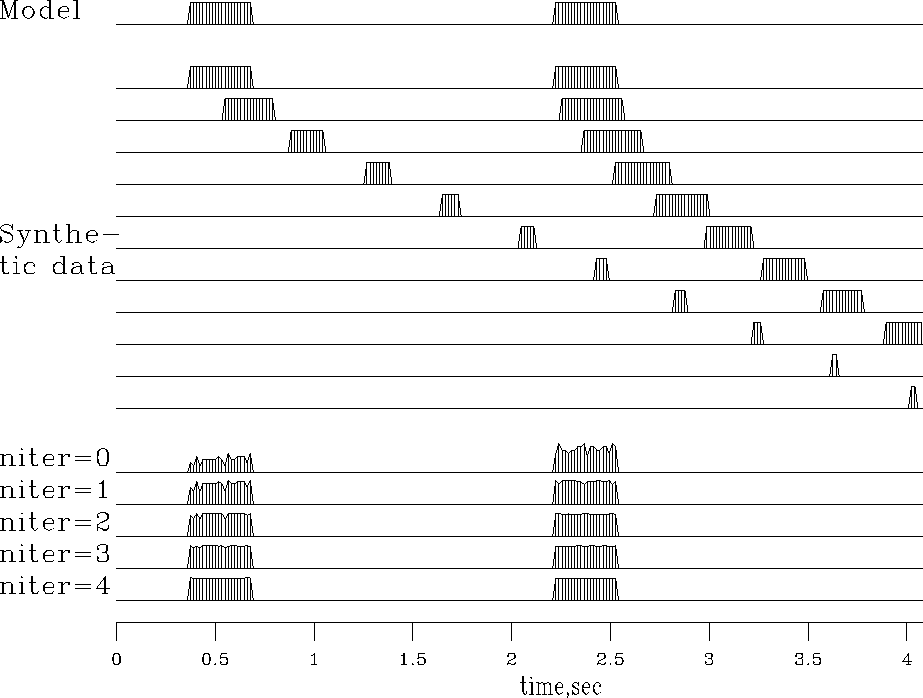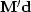Next: MARINE DEGHOSTING Up: Model fitting by least Previous: Preconditioning

# INVERSE NMO STACK

Starting from a hypothetical, ideal, zero-offset model, Figureshows synthetic data and the result of adjoint modeling (back projection), which reconstructs an imperfect model. Inversion should enable us to reconstruct the original model. Let us see how back projection can be upgraded towards inversion.

Unfortunately, the adjoint operatordefined by the subroutine nmo1()is not a good operator for seismogram modeling--notice the roughness of the synthetic seismograms in Figure. This roughness is not an inevitable consequence of nearest-neighbor interpolation. It is a consequence of defining the NMO program as a loop over the output space.Instead, we can define inverse NMO as a loop over its output space, which is notbut t. This is done in imo1()and imospray().

 If we plan an upgrade from back projection towards inversion, we must be aware that the accuracy of the original modeling operator can become an issue.

 invstack Figure 1 Top is a model trace. Next are the synthetic data traces,. Then, labeled niter=0 is the stack, a result of processing by adjoint modeling. Increasing values of niter showas a function of iteration count in the regression. (Carlos Cunha-Filho)The new seismograms at the bottom of Figure 1 show the first four iterations of conjugate-gradient inversion. You see the original rectangle-shaped waveform returning as the iterations proceed. Notice also on the stack that the early and late events have unequal amplitudes, but after iteration they are equal, as they began. Mathematically, we can denote the top trace as the model,the synthetic data signals as,and the stack as.The conjugate-gradient algorithm solves the regressionby variation of,and the figure showsconverging to.Since there are 256 unknowns in,it is gratifying to see good convergence occurring after the first four iterations. The CG subroutine used is invstack(), which is just like cgmeth()except that the matrix-multiplication operator matmul()has been replaced by imospray(). Studying the program, you can deduce that, except for a scale factor, the output at niter=0 is identical to the stack.All the signals in Figure 1 are intrinsically the same scale.

# NMO stack by inverse of forward modeling
#
subroutine invstack(  nt,model,nx,gather,rr,t0,x0,dt,dx,slow,niter)
integer it, ix, iter, nt,      nx,                           niter
real    t0,x0,dt,dx,slow, gather(nt,nx), rr(nt,nx), model(nt)
temporary real  dmodel(nt), smodel(nt), dr(nt,nx), sr(nt,nx)
do it= 1, nt
model(it) = 0.0
do it= 1, nt
do ix= 1, nx
rr(it,ix) = gather(it,ix)
do iter = 0, niter {
call imospray( 1,0,slow,x0,dx,t0,dt,nx,nt,dmodel,rr)    # nmo-stack
call imospray( 0,0,slow,x0,dx,t0,dt,nx,nt,dmodel,dr)    # modeling
call cgstep(iter,   nt, model, dmodel, smodel, _
nt*nx, rr,    dr,     sr)
}
return; end


This simple inversion is inexpensive. Has anything been gained over conventional stack? First, though we used nearest-neighbor interpolation, we managed to preserve the spectrum of the input, apparently all the way to the Nyquist frequency. Second, we preserved the true amplitude scale without ever bothering to think about (1) dividing by the number of contributing traces, (2) the amplitude effect of NMO stretch, or (3) event truncation.

With depth-dependent velocity, wave fields become much more complex at wide offset. NMO soon fails, but wave-equation forward modeling offers interesting opportunities for inversion.Next: MARINE DEGHOSTING Up: Model fitting by least Previous: Preconditioning
Stanford Exploration Project
10/21/1998